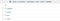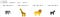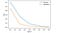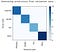# Convolutional Neural Networks with Tensorflow (Safari Dataset)

“Deep Learning is a general term that usually refers to the use of neural networks with multiple layers that synthesize the way the human brain learns and makes decisions. A convolutional neural network is a kind of neural network that extracts features from matrices of numeric values (often images) by convolving multiple filters over the matrix values to apply weights and identify patterns, such as edges, corners, and so on in an image. The numeric representations of these patterns are then passed to a fully-connected neural network layer to map the features to specific classes.”

In this article, we are going to use Tensorflow to solve the classification problem. We are going to use Safari Dataset. I have solved this challenge in my learning so the dataset is not available. However, feel free to use datasets you want such as Fingerprint (SOCOFing) or Breast Cancer Dataset (BreakHis), etc. Search them on kaggle, they should be available. Alright, let’s start!

First up, upgrade the Tensorflow library:

!pip install — upgrade tensorflow

Second, let’s verify we have everything that we need:

`import tensorflowfrom  tensorflow import kerasprint('TensorFlow version:',tensorflow.__version__)print('Keras version:',keras.__version__)TensorFlow version: 2.4.1Keras version: 2.4.0`

Always a good practice to do the above steps! Alright, let’s explore the dataset and see what they look like:

`import numpy as npimport osimport matplotlib.pyplot as pltimport matplotlib.image as mpimg%matplotlib inline# The images are in the data/shapes folderdata_path = 'data/safari/training'# Get the class namesclasses = os.listdir(data_path)classes.sort()print(len(classes), 'classes:')print(classes)# Show the first image in each folderfig = plt.figure(figsize=(12, 12))i = 0for sub_dir in os.listdir(data_path):    i+=1    img_file = os.listdir(os.path.join(data_path,sub_dir))    img_path = os.path.join(data_path, sub_dir, img_file)    img = mpimg.imread(img_path)    img_shape = np.array(img).shape    a=fig.add_subplot(1, len(classes),i)    a.axis('off')    imgplot = plt.imshow(img)    a.set_title(img_file + ' : ' + str(img_shape))plt.show()`

The dataset is placed in the following directory (my local system):Dataset!

That means, elephant, giraffe, lion, and zebra folder exists in the training directory. We have to select the images from these directories and pre-process them to fed into the CNN model. Let’s see the dataset visualization by using the above code:Four Labels (Classifications Right?)

Now, we are going to pre-process the dataset so that it is ready to train in the CNN model that we are going to initialize later!

`# Data Pre-processingfrom tensorflow.keras.preprocessing.image import ImageDataGenerator# setting the image sizeimg_size = (128, 128)batch_size = 30# Setting normalization and training and validation setdatagen = ImageDataGenerator(rescale=1./255,                            validation_split=0.3) # that means 30% of the data is left for validation purpose!# Loading the training dataset from the directorytrain_gen = datagen.flow_from_directory(data_path,                                   target_size=img_size,                                   batch_size=batch_size,                                   class_mode='categorical',                                   subset='training')# loading the validation dataset from the directoryval_gen = datagen.flow_from_directory(data_path,                                   target_size=img_size,                                   batch_size=batch_size,                                   class_mode='categorical',                                   subset='validation')classnames = list(train_gen.class_indices.keys())`

In Tensorflow, ImageDataGenerator is used to process the image dataset! We have set the image size and batch size. We are going to set all the images by 128x128 pixels and feed 30 batches of data in each training. Using these parameters, we have used “flow_from_directory” to generate the training and validation data from the directories. So, training data means, we are going to use these data to learn the model, and “validation dataset is a sample of data held back from training your model that is used to give an estimate of model skill while tuning model’s hyperparameters”.

Next up, let’s check the features and labels:

`# let's check the shapes of the training datasettrain_gen.image_shape# that means 3 sample of the data with 128x128 pixels will enter the CNN Model: (128, 128, 3)Labels:classnames: ['elephant', 'giraffe', 'lion', 'zebra']`

Now, we know about the features and labels. Also, we have a training and validation set. Let’s create the CNN model.

`# Building the CNN Modelfrom tensorflow.keras.models import Sequentialfrom tensorflow.keras.layers import Conv2D, MaxPooling2D, Dropout, Flatten, Dense# we are going to work with sequential modelmodel = Sequential()# The input layer accepts an image and applies a convolution that uses 32 6x6 filters model.add(Conv2D(32, (6, 6), input_shape=train_gen.image_shape, activation='relu'))model.add(MaxPooling2D(pool_size=(2,2)))# We can add as many layers as we think necessary - here we'll add another convolution and max pooling layermodel.add(Conv2D(32, (6, 6), activation='relu'))model.add(MaxPooling2D(pool_size=(2, 2)))model.add(Conv2D(32, (6, 6), activation='relu'))model.add(MaxPooling2D(pool_size=(2, 2)))# A dropout layer randomly drops some nodes to reduce inter-dependencies (which can cause over-fitting)model.add(Dropout(0.2))# Flatten the feature maps model.add(Flatten())# Generate a fully-connected output layer with a predicted probability for each class# (softmax ensures all probabilities sum to 1)model.add(Dense(train_gen.num_classes, activation='softmax'))# With the layers defined, we can now compile the model for categorical (multi-class) classificationmodel.compile(loss='categorical_crossentropy',              optimizer='adam',              metrics=['accuracy'])print(model.summary())`

In the CNN model, we have used three hidden layers along with MaxPooling2D and Model Dropouts. We have also used Conv2D layers to filter the image to extract the features. In the model, you will be able to see Flatten() the model. Before that, everything is used to extract the features from the images, and then finally we have used Adam optimizer, and Categorical Crossentropy to classify the image properly. The most important thing in the CNN model is to specify the input features shape (first layer: (128, 128, 3)) and label shape (last layer: 4). let’s observe the model structure:

`Model: "sequential_1"_________________________________________________________________Layer (type)                 Output Shape              Param #   =================================================================conv2d (Conv2D)              (None, 123, 123, 32)      3488      _________________________________________________________________max_pooling2d (MaxPooling2D) (None, 61, 61, 32)        0         _________________________________________________________________conv2d_1 (Conv2D)            (None, 56, 56, 32)        36896     _________________________________________________________________max_pooling2d_1 (MaxPooling2 (None, 28, 28, 32)        0         _________________________________________________________________conv2d_2 (Conv2D)            (None, 23, 23, 32)        36896     _________________________________________________________________max_pooling2d_2 (MaxPooling2 (None, 11, 11, 32)        0         _________________________________________________________________dropout (Dropout)            (None, 11, 11, 32)        0         _________________________________________________________________flatten (Flatten)            (None, 3872)              0         _________________________________________________________________dense (Dense)                (None, 4)                 15492     =================================================================Total params: 92,772Trainable params: 92,772Non-trainable params: 0_________________________________________________________________None`

Now, it is time to train the model:

`# Train the model over 5 epochs and using the validation holdout dataset for validationnum_epochs = 5history = model.fit(    train_gen,    steps_per_epoch = train_gen.samples // batch_size,    validation_data = val_gen,     validation_steps = val_gen.samples // batch_size,    epochs = num_epochs)Epoch 1/59/9 [==============================] - 17s 2s/step - loss: 1.3095 - accuracy: 0.3583 - val_loss: 0.9090 - val_accuracy: 0.7333Epoch 2/59/9 [==============================] - 14s 2s/step - loss: 0.7083 - accuracy: 0.7767 - val_loss: 0.1498 - val_accuracy: 0.9778Epoch 3/59/9 [==============================] - 14s 2s/step - loss: 0.1613 - accuracy: 0.9447 - val_loss: 0.0535 - val_accuracy: 1.0000Epoch 4/59/9 [==============================] - 13s 1s/step - loss: 0.0765 - accuracy: 0.9812 - val_loss: 0.0188 - val_accuracy: 1.0000Epoch 5/59/9 [==============================] - 13s 1s/step - loss: 0.0286 - accuracy: 1.0000 - val_loss: 0.0059 - val_accuracy: 1.0000`

During the training, we have used 5 epochs which you can see from the output. We have used the batch size to divide the input into 5 epochs which are 9. You can see the validation accuracy from the output which shows the model performances while fine-tuning the parameters. The model produces 100% model accuracy. It makes sense because the dataset is limited! Well, let’s look at loss function projection for both training and validation!

`# let's view the loss throughout the training%matplotlib inlinefrom matplotlib import pyplot as pltepoch_nums = range(1,num_epochs+1)training_loss = history.history["loss"]validation_loss = history.history["val_loss"]plt.plot(epoch_nums, training_loss)plt.plot(epoch_nums, validation_loss)plt.xlabel('epoch')plt.ylabel('loss')plt.legend(['training', 'validation'], loc='upper right')plt.show()`

Output:Loss!!

We can also use the confusion matrix to further verify that our model is suitable in this particular case!

`# Further Verification of model efficiencyimport numpy as npfrom sklearn.metrics import confusion_matriximport matplotlib.pyplot as plt%matplotlib inlineprint("Generating predictions from validation data...")# Get the image and label arrays for the first batch of validation datax_test = val_geny_test = val_gen# Use the model to predict the classclass_probabilities = model.predict(x_test)# The model returns a probability value for each class# The one with the highest probability is the predicted classpredictions = np.argmax(class_probabilities, axis=1)# The actual labels are hot encoded (e.g. [0 1 0], so get the one with the value 1true_labels = np.argmax(y_test, axis=1)# Plot the confusion matrixcm = confusion_matrix(true_labels, predictions)plt.imshow(cm, interpolation="nearest", cmap=plt.cm.Blues)plt.colorbar()tick_marks = np.arange(len(classnames))plt.xticks(tick_marks, classnames, rotation=85)plt.yticks(tick_marks, classnames)plt.xlabel("Predicted Label")plt.ylabel("Actual Label")plt.show()`

And the confusion Matrix would be:Perfect Classifier!

Also, we can save the model to test it with the testing images. Let’s see how to save the model to the local directory!

`# Save the trained modelmodelFileName = 'models/safari_classifier.h5'model.save(modelFileName)del model  # deletes the existing model variableprint('model saved as', modelFileName)model saved as models/safari_classifier.h5`

Later on, we can reload the model and test it with the new images! The benefit is that we don’t have to retrain the CNN model again! More about saving and loading the model can be found here. Well, let’s leave that part up to you! Go have fun with the CNN model!

Cheers! 😃

Data Scientists / Full Stack Deep Learning

## More from Mahedi Hasan Jisan

Data Scientists / Full Stack Deep Learning